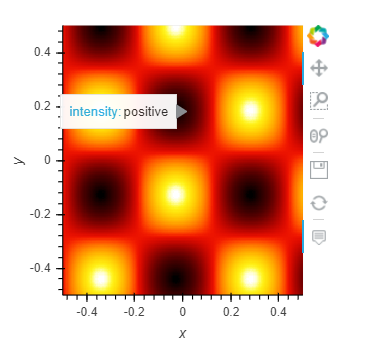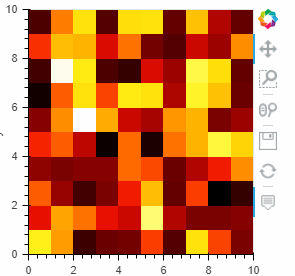# Custom Image HoverTool with mask labels

Hi all,

Could anyone provide some guidance on how to show the x, y coordinates, pixel intensity and label based off of an external segmentation mask (e.g. background = 0, dog = 1, cat = 2, etc.) when hovering over a pixel in an image?

Similar to this:

The solution to your problem is similar to what I showed. The trick is creating your own custom function and linking the z parameter to a new function that you write. Here is an example:

``````import numpy as np
import holoviews as hv
from holoviews import opts
hv.extension('bokeh')
from bokeh.models import HoverTool
from bokeh.models import CustomJSHover
ls = np.linspace(0, 10, 200)
xx, yy = np.meshgrid(ls, ls)

MyCustomZ = CustomJSHover(code='''
var value;
var modified;
if (value>0)
{modified="positive";}
else
{modified="negative";}
return modified.toString();''')

MyHover1 = HoverTool(
tooltips=[
( 'intensity', '@image{custom}'),
],
formatters={
'@image' : MyCustomZ,
},
point_policy="follow_mouse"
)

img = hv.Image(np.sin(xx)*np.cos(yy)).opts(tools=[MyHover1])
img
``````

The output looks like this:The cavate is that the image intensity - the the z value, is called image so the name of the parameter in the hover tool must be `@image`.

Hopefully this example is good enough to resolve your problem.

Not exactly. I want to use an external mask. So it’s either use an Overlay of the image and mask, in which case I have two separated HoverTools and can’t seem to distinguish between the two when using ATimage once joined. Or, I need to add both ATx and ATy to the same custom JS hover, which I can’t quite figure out how to do.

The ideal circumstance would be something like

CustomHover = HoverTool(
tooltips=[
( ‘(x, y)’, ‘(\$x, \$y)’),
(‘Intensity’, ‘ATimage.image’),
],
formatters={
‘(x, y)’ : ‘numeral’,
‘Intensity’: ‘numeral’,
‘Label’: ‘numeral’,
},
point_policy=“follow_mouse”
)

img = hv.Image(img_array, label=“image”)

AT = at symbol

If you can use Raster instead of Image (else the conversion of x, y into indices is a little bit more complex) you can try this:

``````import numpy as np
import holoviews as hv
from bokeh.models import HoverTool, CustomJSHover, ColumnDataSource

hv.extension('bokeh')

hv.opts.defaults(hv.opts.Raster(invert_yaxis=True)) # cf https://github.com/holoviz/holoviews/issues/4666

data = np.random.rand(10,10)
mask = np.random.choice(('dog', 'cat'), size=10*10, replace=True).reshape(10,10)
MyCustomZ = CustomJSHover(args={"cds":cds}, code='''
const idx = Math.floor(special_vars.x)
const idy = Math.floor(special_vars.y)

MyHover1 = HoverTool(
tooltips=[
('x', "\$x"),
('y', "\$y"),
('value', '@image'),
( 'Animal', '@image{custom}'),
],
formatters={
'@image' : MyCustomZ,
},
point_policy="follow_mouse"
)
hv.Raster(data).opts(hv.opts.Raster(tools=[MyHover1]))
``````Perfect, thank you!